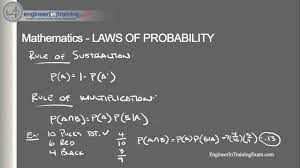Immersing oneself in the world of probabilities enables uncertainty to be quantified. Probability attempts to draw us closer to a place of certainty, where solving problems becomes easier. Similarly, business decisions are more objective and likely to be effective. Previously, we established the probability of an event happening is always 1 or 100%. Conversely, the probability of it not happening is 0. We also talked about the three ways of assigning probability which are the classical, relative frequency, and subjective methods. The focus of this write-up is on the rules (otherwise called law) of probability.

Laws of Probability

There are two main laws in probability- the addition and multiplication law.

However, for a better understanding of the addition rule, the following concepts need to be taken into context.

1. Addition law. This rule is used to quantify the probability that at least one event happens. Simply put, in an experiment, there may be multiple possible events and the addition rules describes this outcome under two scenarios. One is the probability for two mutually events happening. The other is the probability of two non-mutually exclusive events happening. Thus, the addition rule quantifies the probability that at least one of the two events occurred.
• Sample space. This is simply the total number of possible events
• Event. This describes the different possible outcomes of an experiment.
• Mutually exclusive events. Here, two events cannot occur at the same time. For example, when you flip a coin, it is either a Head or a Tail that emerges.
• Non-mutually exclusive events. This implies that a degree of overlap exists between two events.
• Independent events. The occurrence of one event remains unaffected by the occurrence of another event.

The formula to calculate the probability of two events A and B, where and denotes an intersection is

P (A U B) = P(A) + P(B) – P (A B)

This is used for non-mutually exclusive events

Where:

P (A U B) = Probability of A or B occurring

P(A)= Probability of Event A occurring

P(B) =Probability of Event B occurring

P (A ∩ B) =Probability of A and B occurring together

For mutually exclusive events, P (A ∩ B) is zero because A and B cannot occur at the same time. Thus, the formula would be rewritten as P (A U B) = P(A) + P(B)

• Multiplication Law. This law states that the probability of two events occurring simultaneously can be calculated using the known probabilities of each event happening on its own.

For independent events, theprobability is computed as P(A B) = P(A) * P(B)

While independent events are unaffected by each other, dependent events affect each other. For instance, drawing a green marble from a bowl without replacing it will impact the possibility of drawing another green marble. This gives rise to the concept of conditional probability.

For dependent events, the probability isP(B|A) = P(A B)/P(A)

Where:

P(B|A): the probability of B happening given A has happened

P(A ∩ B): the probability of A and B happening together

P(A): the probability of A happening

In conclusion, the addition rule is used to compute the probabilities for at least one event occurring such as in mutually exclusive events. While the multiplication law calculates joint probabilities for independent or dependent events. In subsequent blogs, we will continue to unpack the world of probabilities.

#EMBA27## Understanding the Balance Sheet and the Income Statement: A Guide for Business Managers #MMBA5

in